# vs.eyeandcontacts.com

## Chapter 4 Practical Geometry Exercise 4.3

Question 1: Construct the following quadrilaterals.
MO = 6 cm
OR = 4.5 cm
∠M = 60°
∠O = 105°
∠R = 105°
PL = 4 cm
LA = 6.5 cm
∠P = 90°
∠A = 110°
∠N = 85°
HE = 5 cm
EA = 6 cm
∠R = 85°
OK = 7 cm
KA = 5 cm

i)
Given
PL = 4 cm
LA = 6.5 cm
∠P = 90°
∠A = 110°
∠N = 85°

Rough figure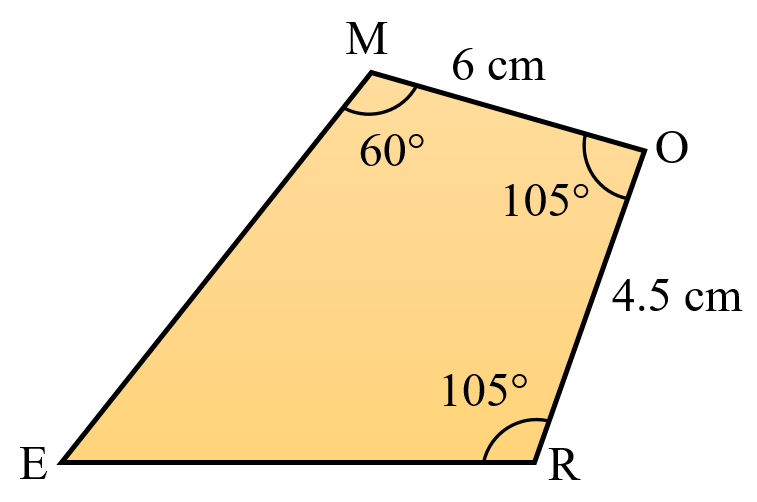Actual figure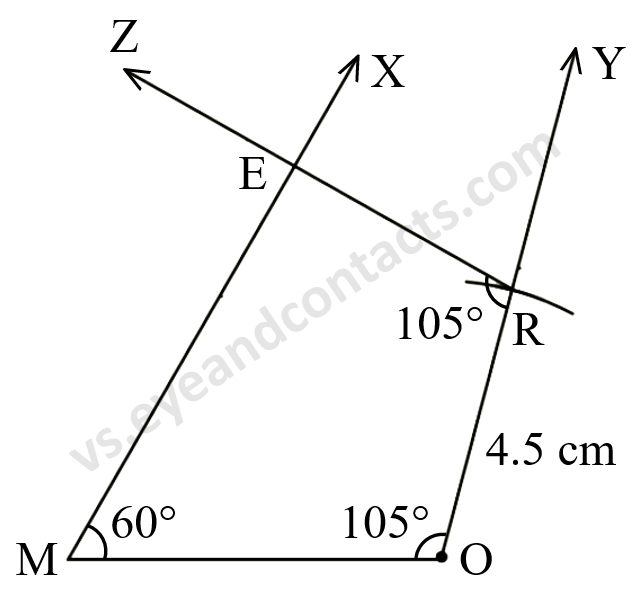Steps of Construction
1. Draw line segment MO = 6 cm.
2. Make ∠OMX = 60°.
3. Make ∠MOY = 105°.
4. With O as centre, radius 4.5 cm draw an arc to cut ray OY at R.
5. Make ∠ORZ = 105°.
6. Let ray MX and ray RZ intersect at E.
7. MORE is the required quadrilateral.

ii)
Given
PL = 4 cm
LA = 6.5 cm
∠P = 90°
∠A = 110°
∠N = 85°

Rough figure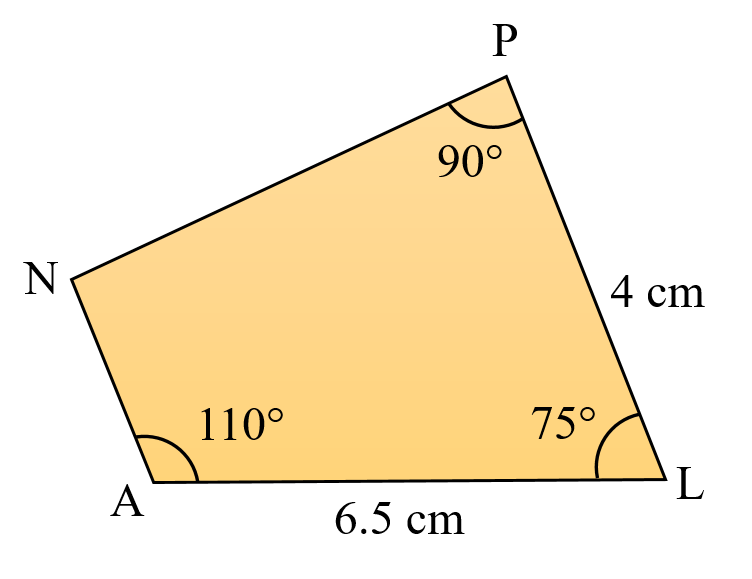Angle sum property of quadrilateral = 360°
= 85° + 90° + 110° + x = 360°
= 285° + x = 360°
= x = 360° - 285°
= x = 75°

Actual figure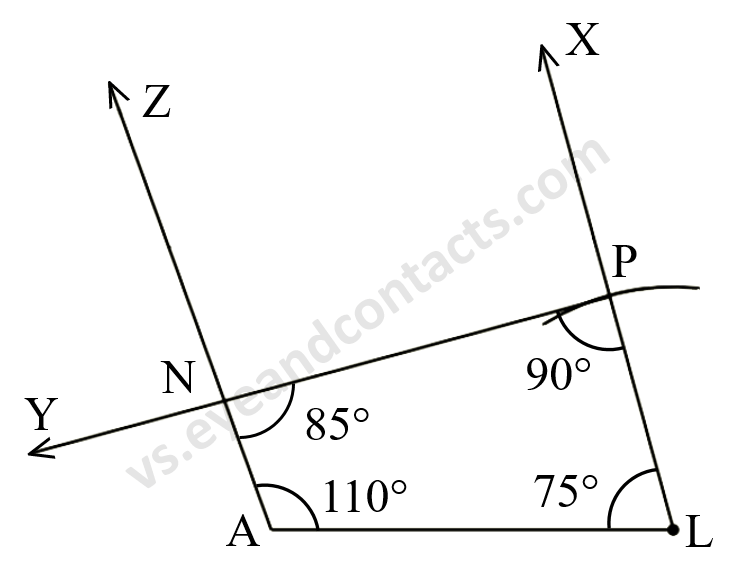Steps of Construction
1. Draw line segment AL = 6.5 cm.
2. Make ∠LAZ = 110°
3. Make ∠ALX = 75°.
4. With L as centre, radius 4 cm draw an arc to cut ray XL at P.
5. Make ∠LPX = 90°.
6. Let ray AX and ray PY intersect at N.
7. PLAN is the required quadrilateral.

iii)
Given
HE = 5 cm
EA = 6 cm
∠R = 85°

Rough figure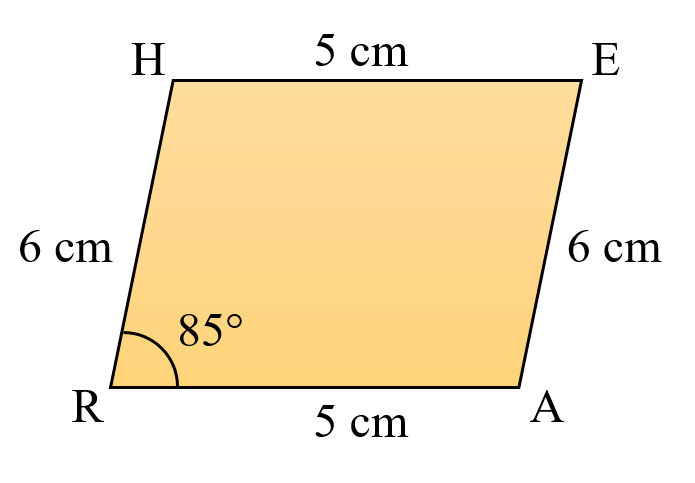∠E = 85° (Opposite angles are equal)
Angle sum property of quadrilateral = 360°
= 85° + x + 85° + x = 360°
= 170° + 2x = 360°
= 2x = 360° - 170°
= 2x = 190°
= x = 190/2
= x = 95°

Actual figure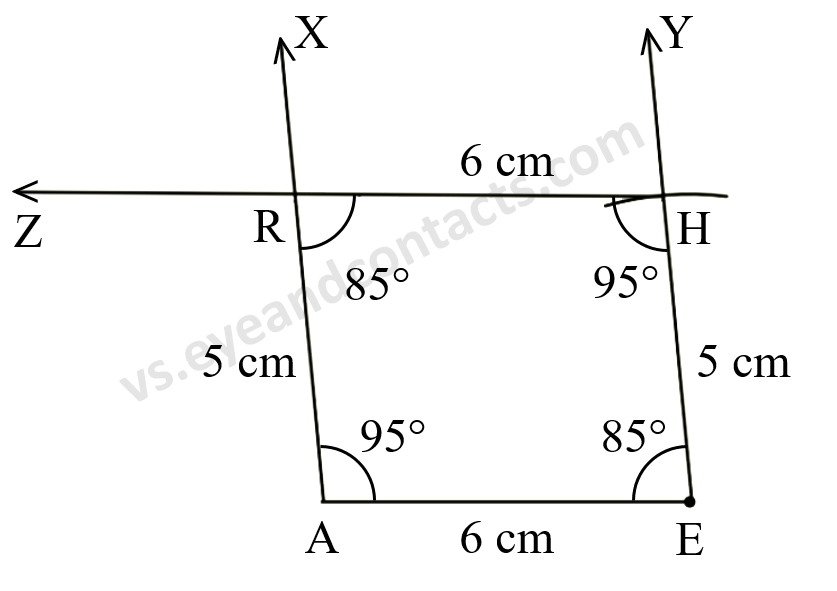Steps of Construction
1. Draw line segment AE = 6 cm.
2. Make ∠XAE = 95°.
3. Make ∠YEA = 85°.
4. With E as centre, radius 5 cm draw an arc to cut ray EY at H.
5. Make ∠EHZ = 95°.
6. Let ray AX and ray HZ intersect at R.
7. HEAR is the required quadrilateral.

iv)
Given
OK = 7 cm
KA = 5 cm

Rough figure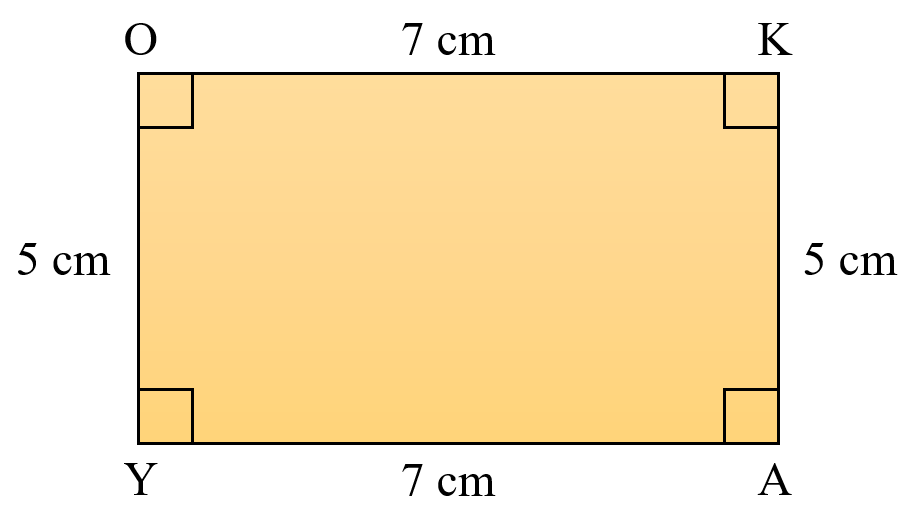Actual figure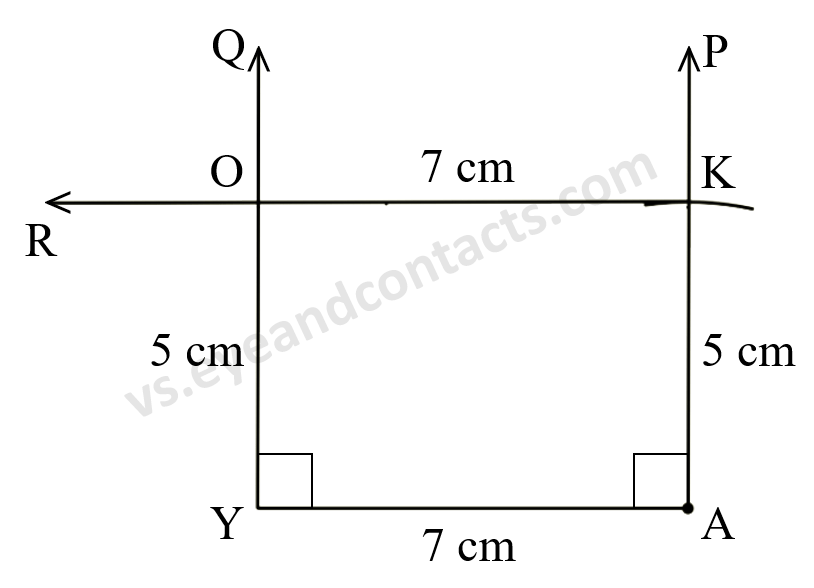Steps of Construction
1. Draw line segment YA = 7 m.
2. Make ∠QYA = 90°.
3. Make ∠PAY = 90°.
4. With A as centre, radius 5 cm draw an arc to ray AP at K.
5. Make ∠AKR = 90°.
6. Let ray QY and ray KR intersect at O.
7. OKAY is the required quadrilateral.